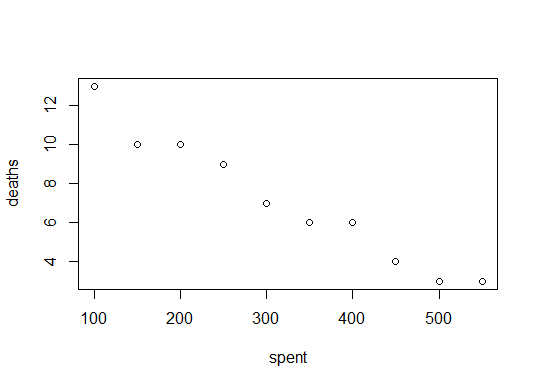# [Solved] Determine if data is related in R [closed]

Lets assume that

``````set.seed(44)
deaths<- 10:1 + sample.int(3, 10, replace = T)
``````

and

``````spent<- seq(100, 550, by = 50 )
``````

The very first thing you want to do when you get your data is literally to look at it. This can be done relatively painlessly with

``````plot(spent, deaths)
``````

which yieldsSo it looks like the more we spend, the less deaths there are. That makes sense. But how can we quantify that statement. Using `cor()` will give us the correlation between the two variables `spent` and `deaths`.

``````cor(spent, deaths)
#  -0.9809581
``````

So it looks like they are very strong (and negatively correlated.) One other simple method (that is closely related to `cor()`) is to fit a linear model.

``````model<- lm(deaths~spent)
``````

The `summary()` call yields a lot of useful information about the model you just fit, the interpretation of which is beyond the scope of this post, but can be readily found with some quick Googling.

``````summary(model)

#Call:
#lm(formula = deaths ~ spent)

#Residuals:
# Min       1Q   Median       3Q      Max
#-0.89697 -0.51515 -0.05758  0.46364  1.01818

#Coefficients:
#            Estimate Std. Error t value Pr(>|t|)
#(Intercept) 14.151515   0.539649   26.22 4.80e-09 ***
#spent       -0.021697   0.001519  -14.29 5.62e-07 ***
#---
#Signif. codes:  0 ‘***’ 0.001 ‘**’ 0.01 ‘*’ 0.05 ‘.’ 0.1 ‘ ’ 1

#Residual standard error: 0.6898 on 8 degrees of freedom
#Multiple R-squared:  0.9623,   Adjusted R-squared:  0.9576
#F-statistic: 204.1 on 1 and 8 DF,  p-value: 5.622e-07
``````

solved Determine if data is related in R [closed]